• Matlab绘图模板

千次阅读 2019-04-11 21:19:49
Matlab绘图高级部分【转】 http://www.cnblogs.com/jeromeblog/p/3396494.html 你好！ 这是你第一次使用 Markdown编辑器 所展示的欢迎页。如果你想学习如何使用Markdown编辑器, 可以仔细阅读这篇文章，了解一下...

1.matlab官网提供的绘图模板

代码也均可在官网找到。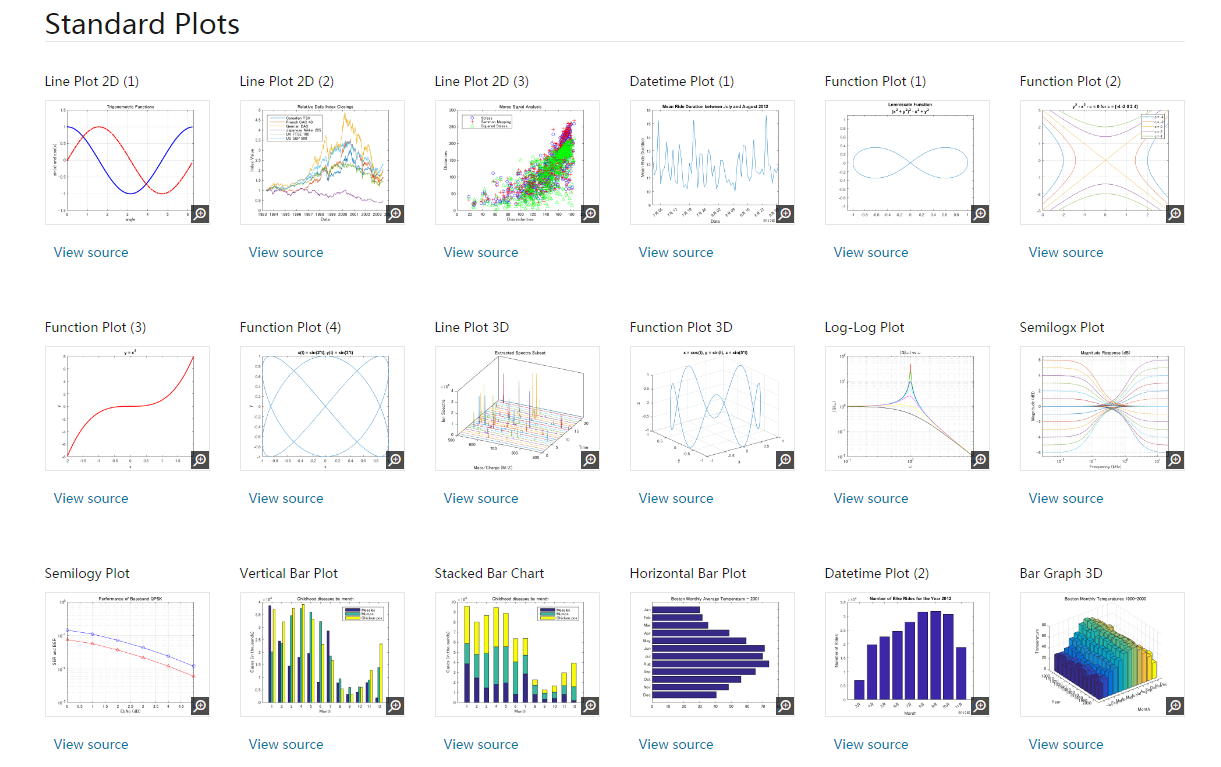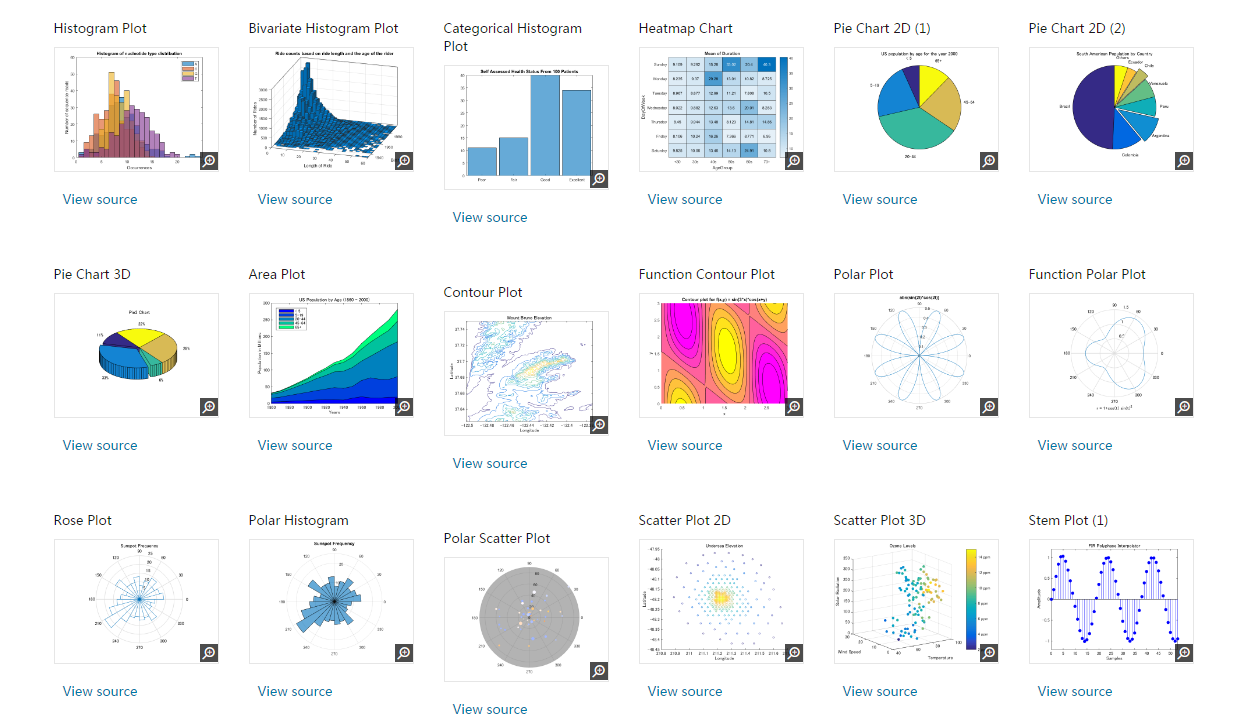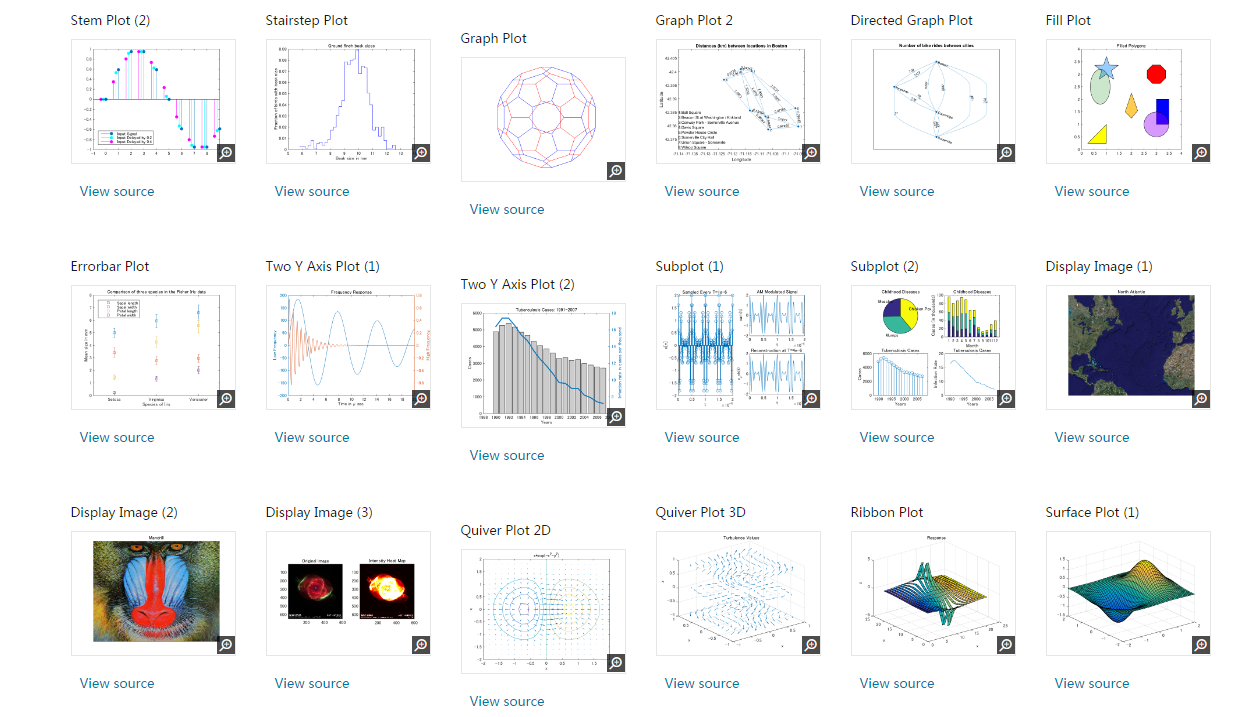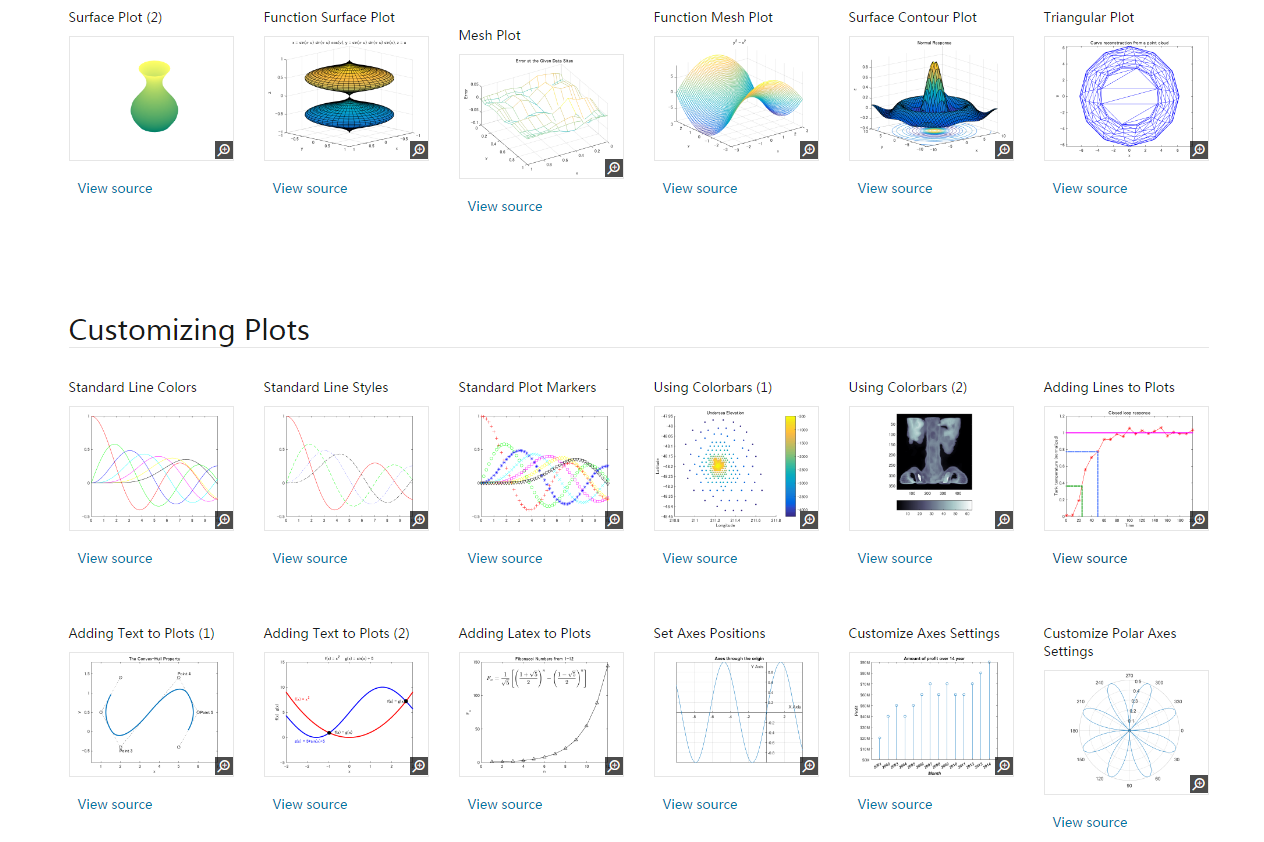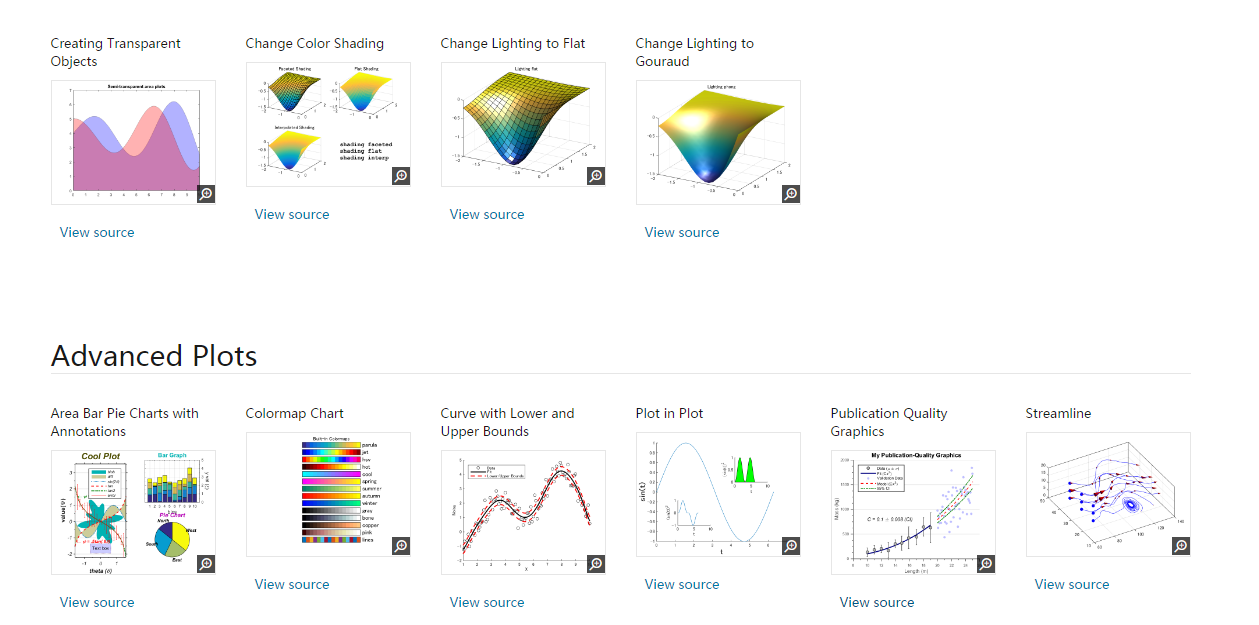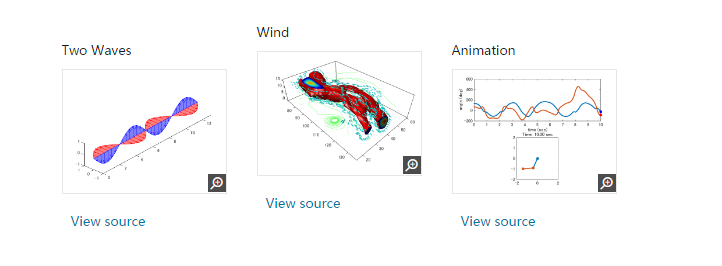2.gramm工具箱

matlab画图的锯齿风再次被某长江大佬嫌弃了，这里推荐一款类似ggplot2的MATLAB工具箱，gramm工具箱【1】下载地址
这里不一一的列举图片效果了，简单看下可视化效果。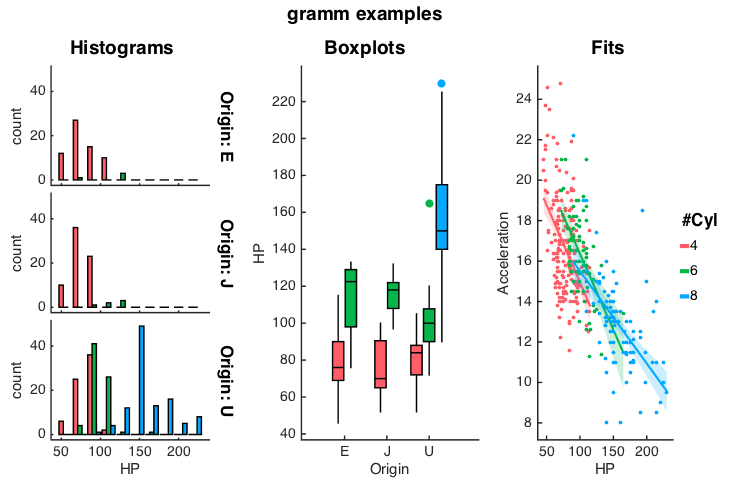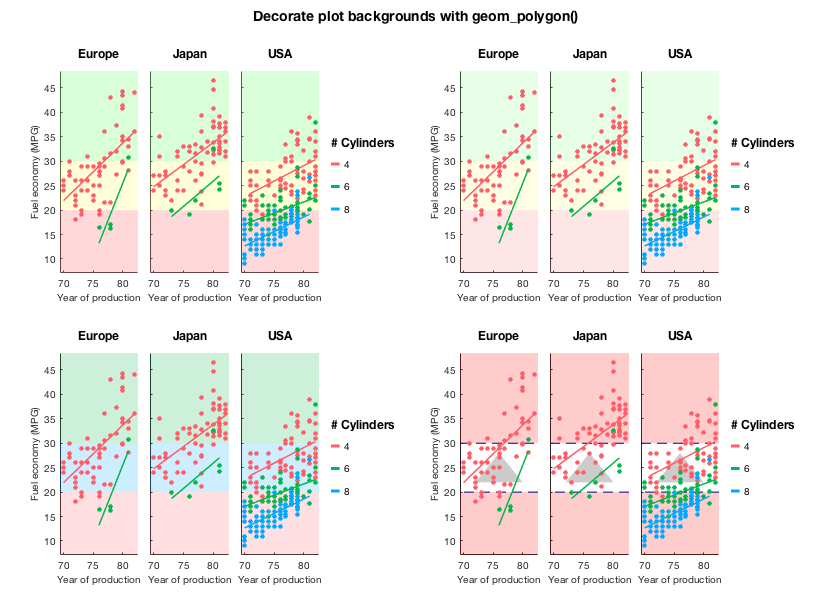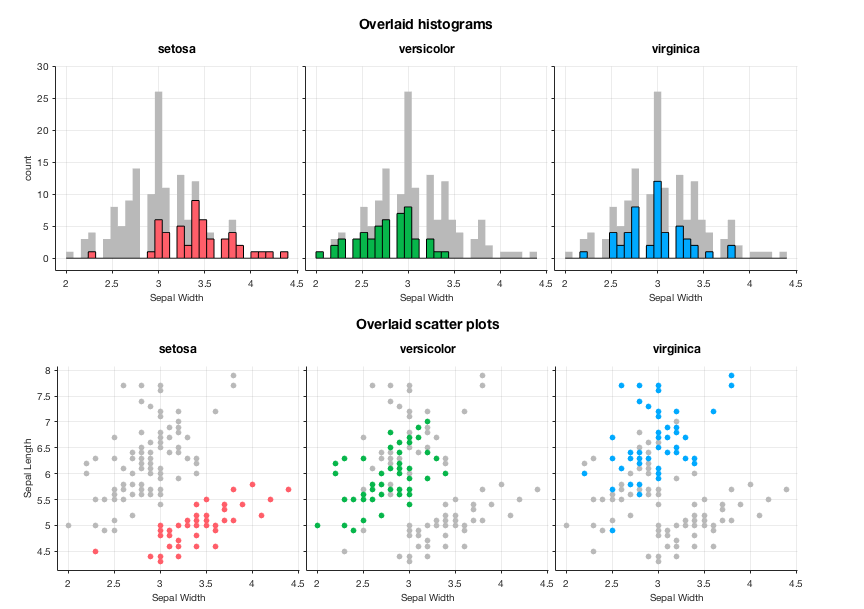3 PlotHub

类origin的matlab神器

参考文献

【1】Morel P . Gramm: grammar of graphics plotting in Matlab[J]. The Journal of Open Source Software, 2018, 3(23):568.
【2】gramm代码改进

展开全文• 经常用到matlab画图功能，最常用的是plot，其他一些图每次用到都要重新百度查询，这里做一个总结，方便查看。 1.plot plot(x,y)可以直接画线，散点图，其中x，y为长度相同的向量； plot(x,y,'bo-')，实线，蓝色...

经常用到matlab的画图功能，最常用的是plot，其他一些图每次用到都要重新百度查询，这里做一个总结，方便查看。

1.plot

plot(x,y)可以直接画线，散点图，其中x，y为长度相同的向量；

plot(x,y,'bo-')，实线，蓝色，点的标记为“o”；

plot(x,y,'bo')，散点，蓝色，点的标记为“o”；

2.scatter

scatter(x,y)，标记为蓝色圆圈的散点图；

scatter（X，Y，S）S确定每个标记的面积。 S可以是与X和Y相同长度的矢量或标量。如果S为空，则使用默认大小，如果S是标量，则MATLAB绘制所有标记的大小相同。如果S是矢量，则分别对应为X、Y所确定圆的大小；

scatter（X，Y，S，C）C确定每个标记的颜色。当C是与X和Y相同长度的向量时，C中的值被线性映射到当前色彩映射中的颜色。当C是1×3矩阵时，它将标记的颜色指定为RGB值。

3.line

line([x1 x2],[y1 y2])画直线，x1、x2分别为起点、终点的横坐标，y1、y2分别为起点、终点的纵坐标；

除此之外的一些图像修饰

1. 添加标题：

title('标题')

2. 添加图例

legend('图例1','图例2')

3.添加标签

xlabel('x轴标签')

ylabel('y轴标签')

4.添加文字描述

text([x,y],'文字描述')在指定位置(x,y)添加描述

5.颜色和线条

b    blue   蓝              .     point               -     solid
g    green  绿            o     circle              :     dotted
r    red    红                x     x-mark            -.    dashdot
c    cyan   青             +     plus                --    dashed
m    magenta 品红   *     star
y    yellow 黄            s     square
k    black  黑             d     diamond
w    white  白            v     triangle (down)
^     triangle (up)
<     triangle (left)
>     triangle (right)
p     pentagram 五角星
h     hexagram  六角星

一张图上多条线用hold on

一张图上多个子图用subplot

subplot(2,2,1)；plot(x,y)

两行两列的子图，第一个位置plot画图

展开全文• 1、使用matlab绘图函数，绘制好图形以后，为了丰富图形的内涵，还必须对图形添加标题、坐标轴标签、文字说明、图例、辅助线、指示线等。详情见下： 2、视角与色彩控制 1）、视角控制 2）、色彩控制 ...

一、知识点

1、使用matlab绘图函数，绘制好图形以后，为了丰富图形的内涵，还必须对图形添加标题、坐标轴标签、文字说明、图例、辅助线、指示线等。详情见下：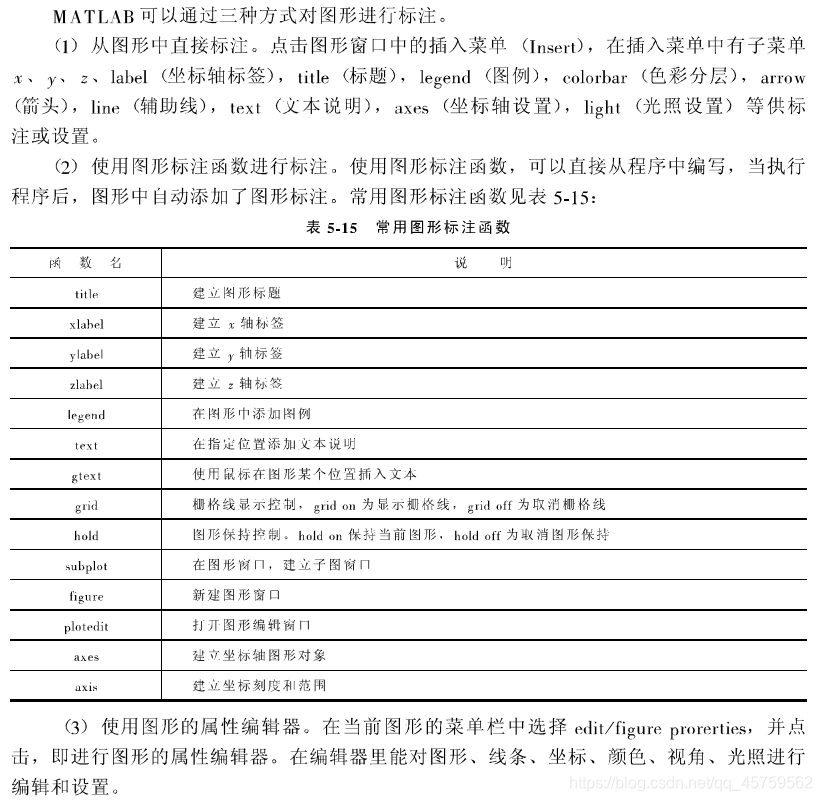2、视角与色彩控制

1）、视角控制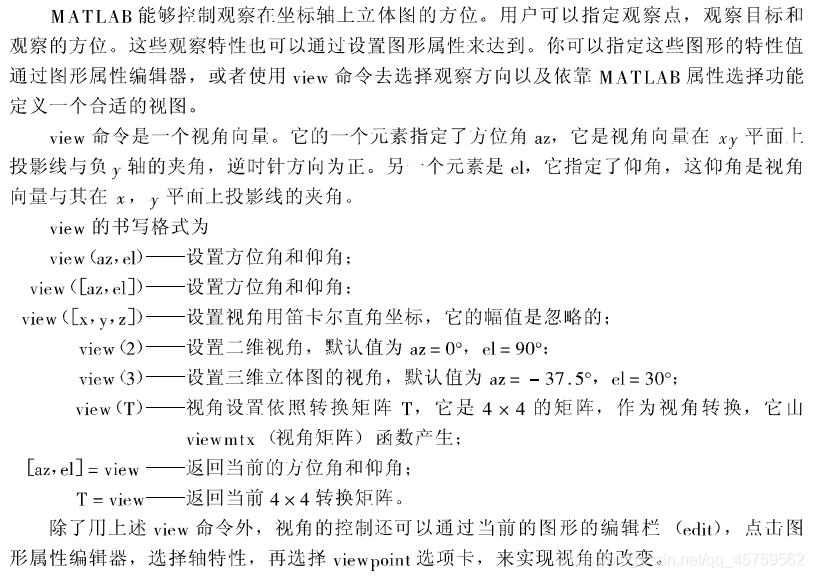2）、色彩控制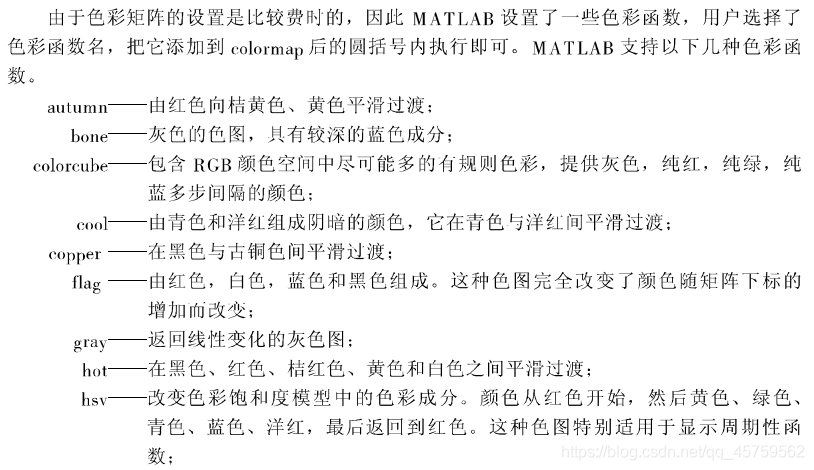二、实例演练

1、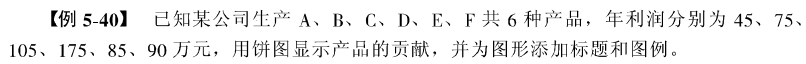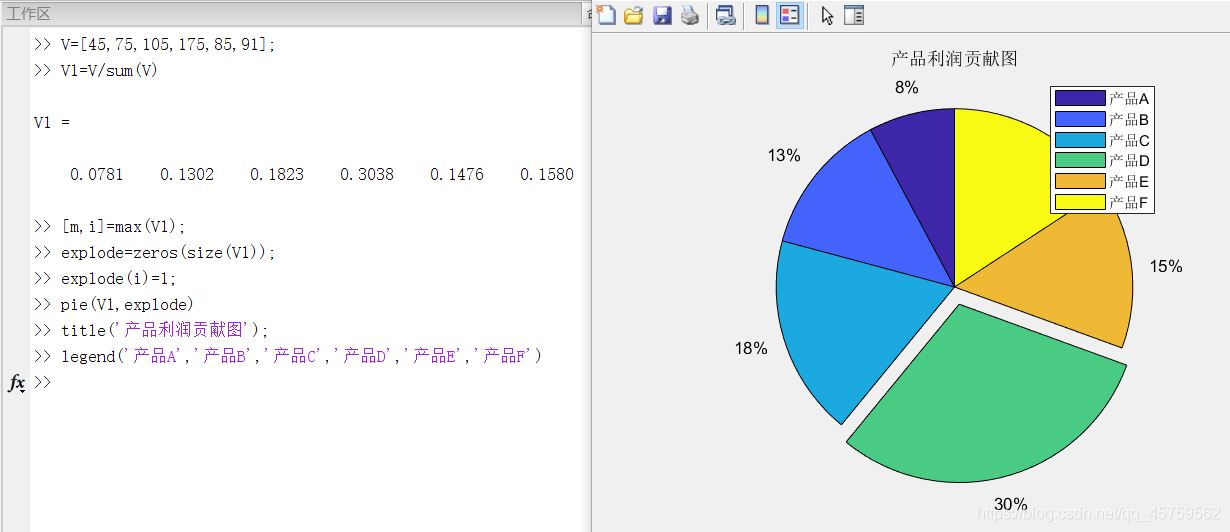2、

1）绘制默认视角下的带有缺口的衰减正弦波

2）将1）中图形的视角改变为10.5°和30°【提示：使用view命令】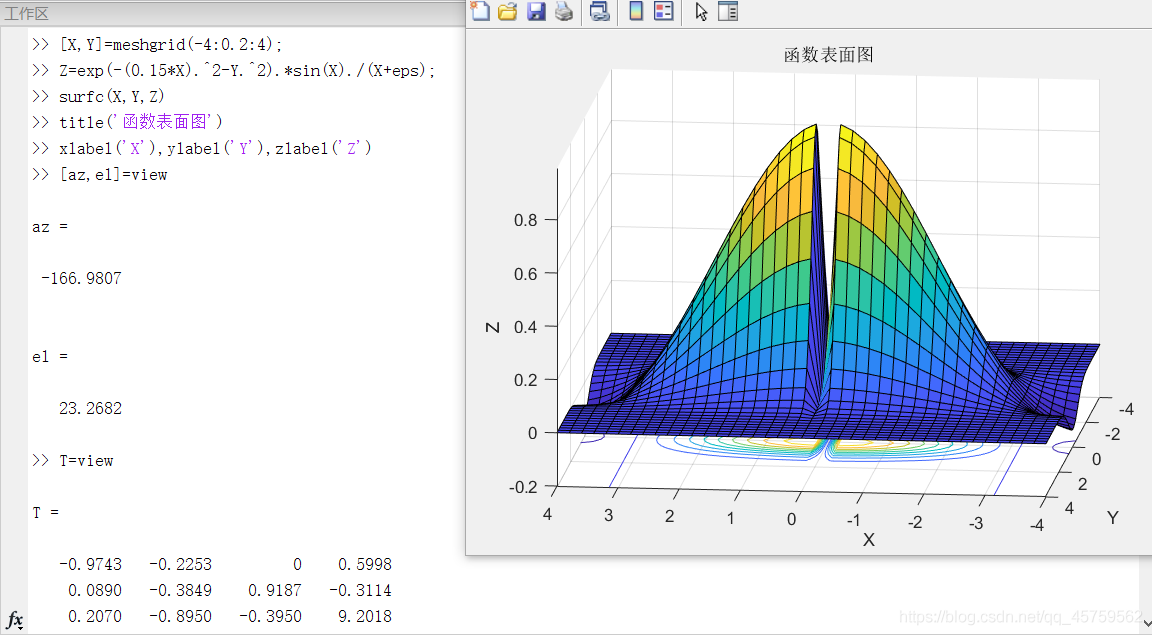三、总结思考

1、本章有大量的图形编辑和设置函数，是MATLAB的一个重点。要不断回顾，复习。

2、MATLAB较C++的不同点是：MATLAB更方便一些，这在声明变量和引用函数时有很大的体现。

展开全文• mfc 调用matlab 画图，（matlab版本是7.0）.封装成类了 配置方法参考 http://blog.csdn.net/woqunokia/article/details/53927702
• 【Matlab】MATLAB绘图

万次阅读 多人点赞 2018-06-29 17:18:39
专题四 MATLAB绘图 绘图的目的是使数据可视化。 一 二维曲线 1. 函数plot() 在MATLAB中，函数plot()是最基本的绘图函数，利用它可以绘制出不同的二维曲线。函数plot()的基本用法： plot(x, y) % 其中，x和y...

专题四    MATLAB绘图

绘图的目的是使数据可视化。

一    二维曲线

1. 函数plot()

在MATLAB中，函数plot()是最基本的绘图函数，利用它可以绘制出不同的二维曲线。函数plot()的基本用法：

plot(x, y)   %  其中，x和y分别用于存储x坐标和y坐标数据，通常x和y是长度相等的向量

举例：绘制一条折线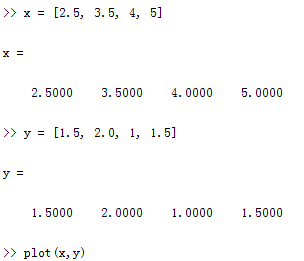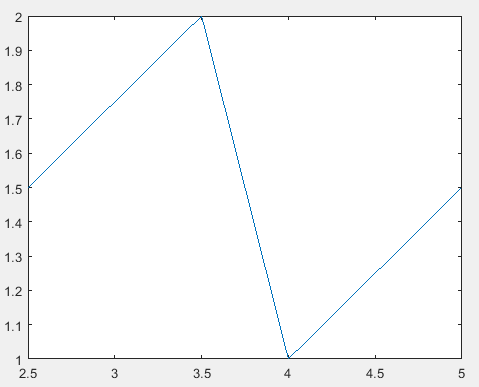向量x的第一个元素和向量y的第一个元素构成了第一个点的坐标，向量x的第二个元素和向量y的第二个元素构成了第二个点的坐标，以此类推。

2. 最简单的plot函数调用格式

plot(x)

• 当x是一个实向量时，以x元素的下标为横坐标，以x元素的值为纵坐标，绘制一条曲线。

举例。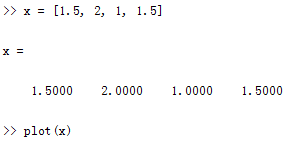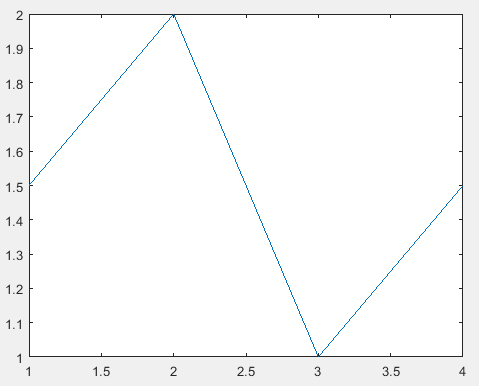• 当函数plot()的参数x是复数向量时，则分别以该向量元素的实部和虚部为横、纵坐标绘制出一条曲线。

举例。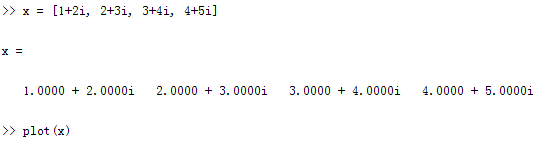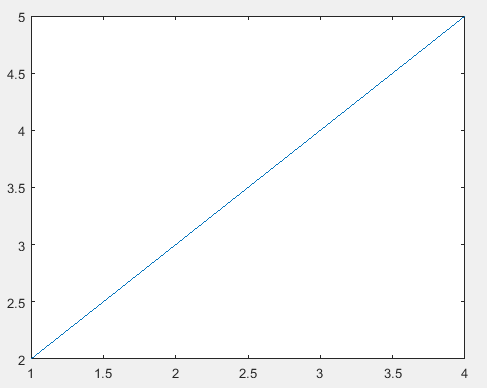3. 函数plot(x,y)参数的变化形式

通常情况下，x和y是长度相等的向量，但是会存在以下一些变化形式：

1. 当x是向量，y是矩阵时，向量x的长度与矩阵y的行数或者列数必须相等

• 如果矩阵y的列数等于x的长度，则以向量x为横坐标，以y的每个行向量为纵坐标绘制曲线，曲线的条数等于y的行数。
• 如果矩阵y的行数等于x的长度，则以向量x为横坐标，以y的每个列向量为纵坐标绘制曲线，曲线的条数等于y的列数。

2. 当x,y是同型矩阵时，以x,y对应列元素为横、纵坐标分别绘制曲线，曲线条数等于矩阵的列数。

4. 含多个输入参数的plot函数

plot(x1, y1, x2, y2, ..., xn, yn)，其中，每一向量对构成一组数据点的横、纵坐标绘制一条曲线，有多少向量对就绘制多少条曲线。

clear; clc;
t1 = linspace(0, 2*pi, 10);
t2 = linspace(0, 2*pi, 20);
t3 = linspace(0, 2*pi, 100);
plot(t1, sin(t1), t2, sin(t2)+1, t3, sin(t3)+2);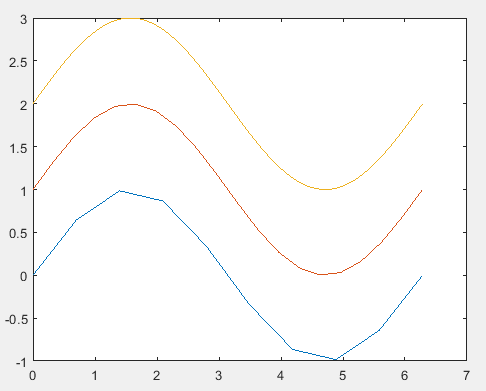5. 含选项的plot函数

plot(x, y, 选项)   % 选项包括线型、颜色、数据点标记等

线型选项有：-（实线）:（虚线）-.（点画线）--（双画线）。当线型选项省略时，默认采用实线

颜色选项有：r（红色）、g（绿色）、b（蓝色）、w（白色）、k（黑色）等。

数据点标记选项有：*（星号）、o（圆圈）、s（方块）、p（五角星）、^（朝上的三角符号）等。当数据点标记选项省略时，默认无数据点标记符号。

6. 函数fplot()

基本用法： fplot(f, lims, 选项)

其中，f代表一个函数，通常采用函数句柄的形式。lims为x轴的取值范围，用二元行向量[xmin, xmax]描述，默认值为[-5, 5]。选项定义与plot函数相同。

举例：采用fplot函数绘制sin(1/x)的图形。

fplot(@(x)sin(1./x), [0, 0.2], 'b');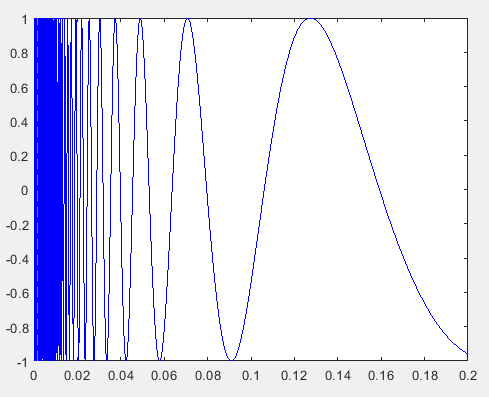双输入函数参数的用法：fplot(funx, funy, tlims, 选项)

其中，funx, funy表示函数，通常采用函数句柄的形式。tlims为参数函数funx, funy的自变量的取值范围，用二元行向量[tmin, tmax]描述，默认值为[-5, 5]。选项定义与plot函数相同。

举例：已知螺旋线的参数方程，绘制曲线。（x = t*sint, y = t*cost）

fplot(@(t)t*sin(t), @(t)t*cos(t), [0, 10*pi], 'b');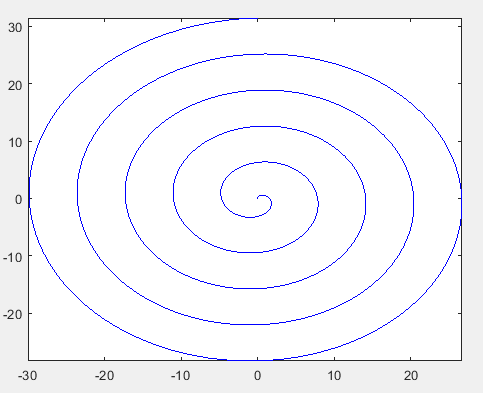------------------------------------------------------------------------------

二    绘制图形的辅助操作

常用的辅助操作有：添加图形标注函数，坐标控制，图形保持，图形窗口的分割等。

1. 添加图形标注函数

• title(图形标题)   % 图形标题需要用字符表示
• xlabel(x轴说明)
• ylabel(y轴说明)
• text(x, y, 图形说明)   % 在指定位置给图形添加说明
• legend(图例1，图例2，…)

1.1 title函数

title函数的基本用法是： title(图形标题)

例如：title('y=sinx')，title({'matlab', 'y=sinx'})（当标题需要多行显示时，采用这种写法。用大括号括起来，标题的每行之间用逗号分隔）

clear; clc;
x = linspace(0, 2*pi, 100);
y = sin(x);
plot(x, y, 'b');
title({'this is a figure','and it''s', 'y=sin(x)'});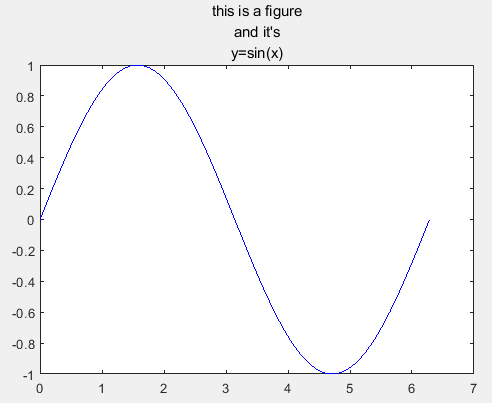title函数中的图形标题文字除了可以使用标准的ASCII字符外，还可以使用LaTex格式控制符。如下所示：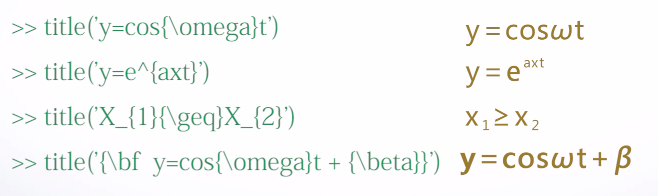其中，\bf表示加粗，此外还有\it表示斜体，\rm表示正体。它们都是对其后的所有字符串起作用。

含属性设置的title函数

title(图形标题，属性名，属性值)

这里的属性名有：Color属性和FontSize属性。Color属性的设置参考plot函数，默认为黑色。FontSize属性，默认的字号为11。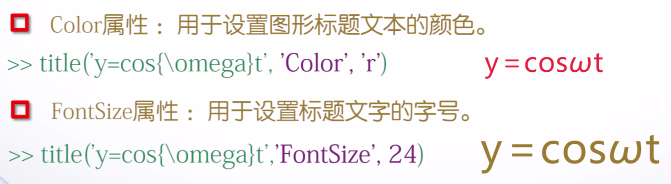1.2 xlabel函数和ylabel函数

调用格式：

• xlabel(x轴说明)
• ylabel(y轴说明)

举例说明。

close; clear; clc;
x = -2*pi : 0.05 : 2*pi;
y = sin(x);
plot(x,y, 'b');
title('y=sin(x)');
xlabel('-2\pi\leqx\leq2\pi'); % 这里的\pi表示π，\leq表示≤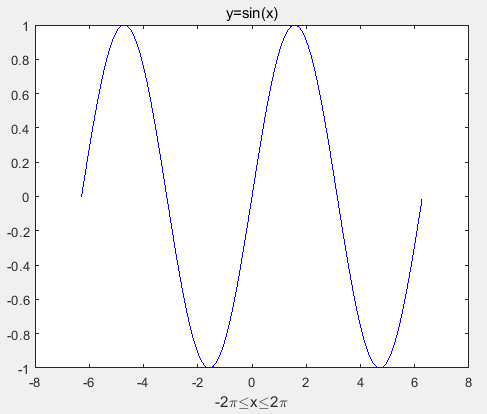1.3 text函数和gtext函数

调用格式如下：

• text(x,y,说明)   % x, y用于设置文本出现的位置
• gtext(说明)   % 用鼠标在figure中自定义地放置标注

举例说明。

close; clear; clc;
x = -2*pi : 0.05 : 2*pi;
y = sin(x);
plot(x,y, 'b');
title('y=sin(x)');
xlabel('-2\pi\leqx\leq2\pi'); % 这里的\pi表示π，\leq表示≤
text(-2*pi, 0, '-2{\pi}');  % 将-2π放置在(-2π,0)的位置处
text(3, 0.28, '\leftarrow sin(x)'); % 在(3,0.28)处用←sin(x)标注，其中\leftarrow表示左箭头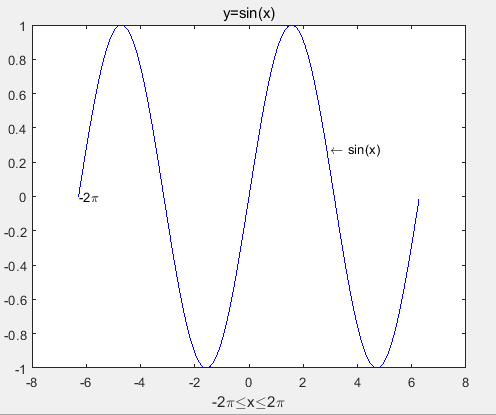1.4  legend函数

调用格式：

• legend(图例1，图例2，…)

举例：绘制不同频率的正弦函数并用图例标注曲线。

close; clear; clc;
x = linspace(0, 2*pi, 100);
plot(x, [sin(x); sin(2*x); sin(3*x)]);
legend('sin(x)', 'sin(2*x)', 'sin(3*x)');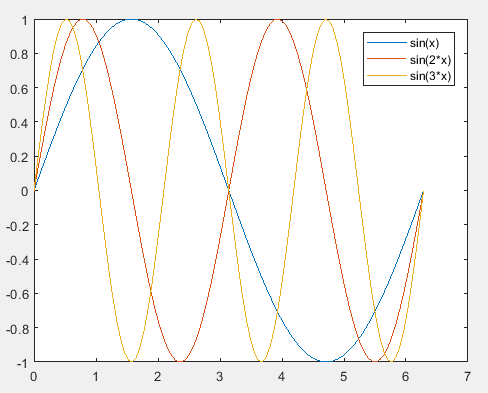2. 坐标控制

2.1  axis函数

调用格式： axis([xmin, xmax, ymin, ymax, zmin, zmax])   % 设置x, y, z轴的坐标范围

举个例子。

close; clear; clc;
axis([-2, 2, -3, 3]);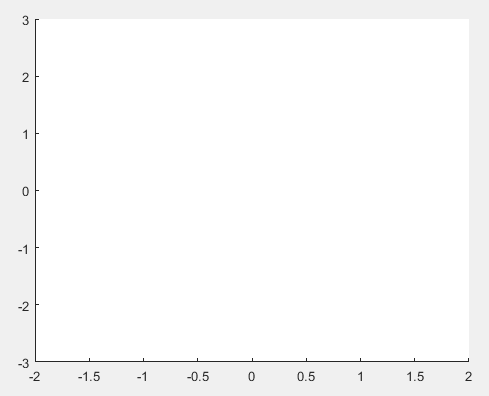axis函数的其他用法：

• axis equal：纵、横坐标轴采用等长刻度
• axis square：产生正方形坐标系（系统默认的是矩形坐标系）
• axis auto：使用坐标轴的默认设置
• axis off：不显示坐标轴
• axis on：显示坐标轴

举个例子。

close; clear; clc;
x = [0,1,1,0,0];
y = [0,0,1,1,0];
plot(x, y);
axis([-0.1, 1.1, -0.1, 1.1]);
axis equal;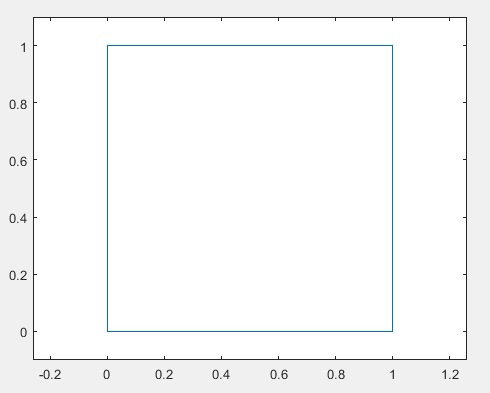2.2 给坐标系加网格和边框

• grid on：显示网格线
• grid off：不显示网格线
• grid：用于在上述两种状态之间进行切换。（比如先是没有网格线，加上语句grid后，会显示网格线，再加一个语句grid后，网格线又不显示了）
• box on：给坐标系加边框
• box off：曲线坐标系的边框
• box：用于在上述两种状态之间进行切换。（与grid的用法相同）

举个例子：绘制sin(x), sin(2x), sin(x/2)的函数曲线并添加图形标注。

close; clear; clc;
x = linspace(0, 2*pi, 100);
y = [sin(x); sin(2*x); sin(x/2)];
plot(x, y);
axis([0, 7, -1.2, 1.2]);
title('不同频率正弦函数曲线');
xlabel('Variable X');
ylabel('Variable Y');
text(2.5, sin(2.5), 'sin(x)');
text(1.5, sin(2*1.5), 'sin(2*x)');
text(5.5, sin(5.5/2), 'sin(x/2)');
legend('sin(x)', 'sin(2*x)', 'sin(x/2)');
grid on;
box on;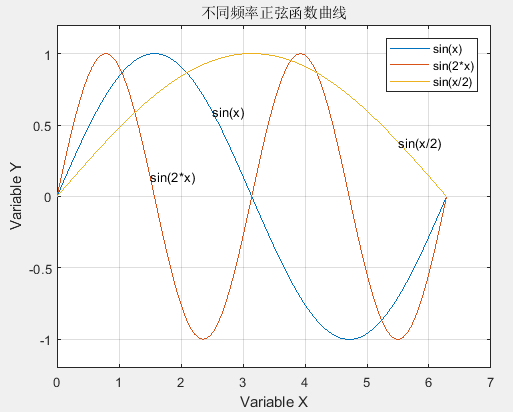2.3 图形保持

• hold on：保持原有的图形（后续操作在该图形上进行）
• hold off：刷新图形窗口
• hold：在上述两种状态之间切换

举个例子：用图形保持功能绘制两个同心圆。

close; clear; clc;
t = linspace(0, 2*pi, 100);
x = sin(t);
y = cos(t);
plot(x, y, 'b');
hold on;
plot(2*x, 2*y, 'r');
axis([-2.2, 2.2, -2.2, 2.2]);
axis equal;
grid on;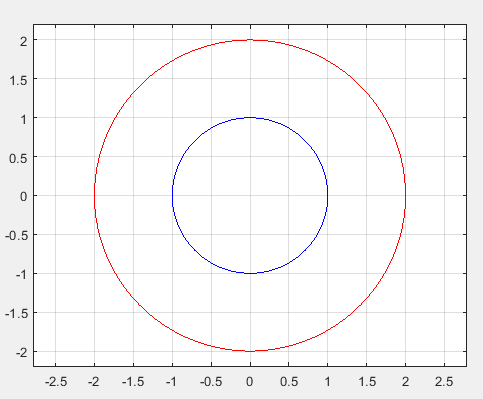2.4  图形窗口的分割

• 子图：同一图形窗口中不同坐标系下的图形称为子图。
• subplot函数

调用格式： subplot(m, n, p)

其中，m和n指定将图形窗口分成m×n个绘图区，p指定当前活动区。

举个例子：灵活地使用图形窗口。

close; clear; clc;
x = linspace(0, 2*pi, 60);
subplot(2, 2, 1);
plot(x, sin(x)-1);
title('sin(x)-1');
axis([0, 2*pi, -2, 0]);
subplot(2, 1, 2);  % 注意这里
plot(x, cos(x)+1);
title('cos(x)+1');
axis([0, 2*pi, 0, 2]);
subplot(4, 4, 3); % 注意这里
plot(x, tan(x));
title('tan(x)');
axis([0, 2*pi, -40, 40]);
subplot(4, 4, 8); % 注意这里
plot(x, cot(x));
title('cot(x)');
axis([0, 2*pi, -35, 35]);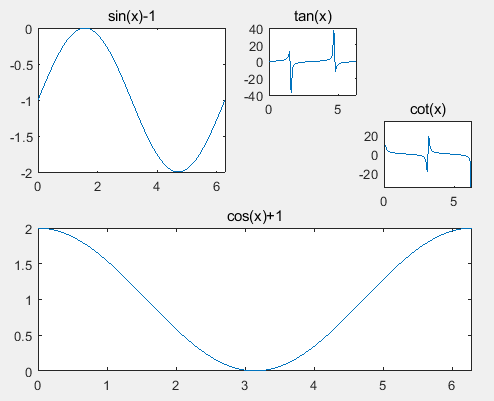------------------------------------------------------------------------------

三    其他形式的二维曲线

1. 其他坐标系下的二维曲线图

1.1 对数坐标图

• semilogx(x1, y1, 选项1, x2, y2, 选项2, ...）  % 半对数坐标函数， x轴为常用对数刻度，而y轴保持常用线性刻度
• semilogy(x1, y1, 选项1, x2, y2, 选项2, ...)    %  半对数坐标函数，y轴为常用对数刻度，而x轴保持常用线性刻度
• loglog(x1, y1, 选项1, x2, y2, 选项2, ...)    % 全对数坐标函数，x轴和y轴均采用常用对数刻度

上述3个函数中，选项的定义与plot函数一致，所不同的是坐标轴的表示。3个函数的调用方法与plot函数相同。

举个例子：绘制1/x的直角线性坐标图和三种对数坐标图。

close; clear; clc;
x = 0 : 0.1 : 10;
y = 1 ./ x;
subplot(2, 2, 1);
plot(x, y);
title('plot(x, y)');
subplot(2, 2, 2);
semilogx(x, y);
title('semilogx(x, y)');
subplot(2, 2, 3);
semilogy(x, y);
title('semilogy(x, y)');
subplot(2, 2, 4);
loglog(x, y);
title('loglog(x, y)');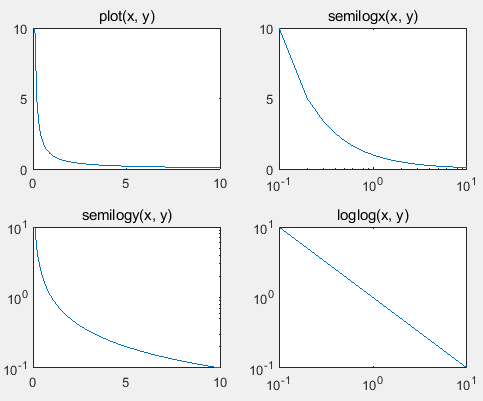1.2 极坐标图

调用格式： polar(theta, rho, 选项)

其中，theta为极角，rho为极径，选项的内容与plot函数相同。

举个例子：按极坐标方程ρ=1-sinθ绘制心形曲线。

close; clear; clc;
t = 0 : pi/100 : 2*pi;
rho = 1 - sin(t);
subplot(1, 2, 1);
polar(t, rho);
subplot(1, 2, 2);
t1 = t - pi/2;
rho1 = 1 - sin(t1);
polar(t, rho1);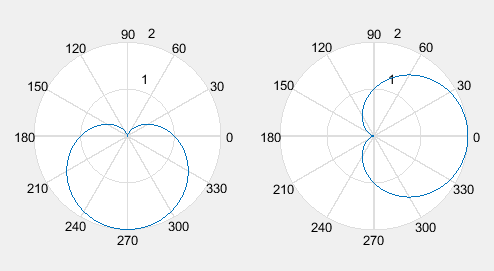2. 统计图

2.1 条形类图形

2.1.1 条形图

条形图的绘制函数有：bar函数绘制垂直条形图，barh函数绘制水平条形图。它们的调用格式相同。

以bar函数为例，其调用格式为： bar(y, style)，其中参数y为数据，选项style用于指定分组排列模式。

• 若y是一个向量，则以每个元素的值作为每个矩形条的高度，以对应元素的下标作为横坐标。若y为矩阵，则以y的每一行元素组成一组，用矩阵的行号作为横坐标，分组绘制矩形条。举个例子：y是一个5阶魔方阵，绘制其矩形条。
close; clear; clc;
A = magic(5);
bar(A);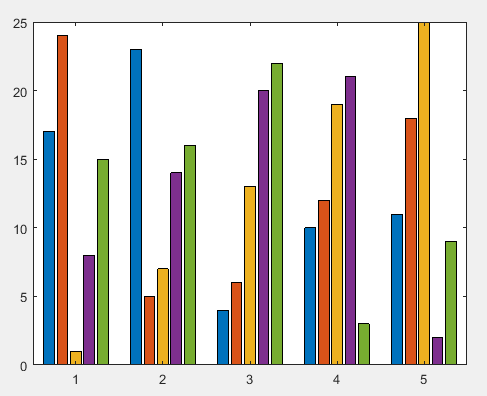选项style用于指定分组排列模式。有“grouped”（簇状分组）、“stacked”（堆积分组）两种取值。默认值是"grouped"。举个例子：绘制分组条形图。

close; clear; clc;
y = [1, 2, 3, 4, 5; 1, 2, 1, 2, 1; 5, 4, 3, 2, 1];
subplot(1, 2, 1);
bar(y);
title('grouped');
subplot(1, 2, 2);
bar(y, 'stacked');
title('stacked');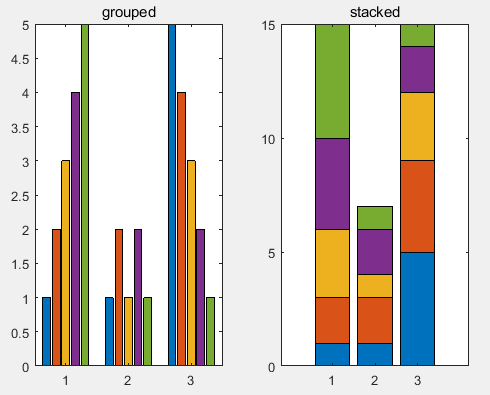此外，bar函数的调用格式还有bar(x, y, style)，其中，x存储横坐标，y存储数据。x是一个向量，y是一个矩阵，向量x的长度要与矩阵y的行数相同。

举个例子。

close; clear; clc;
x = [2016, 2017, 2018];
y = [68, 80, 115, 98, 102; 75, 88, 102, 99, 110; 81, 86, 125, 105, 115];
bar(x, y);
title('Group');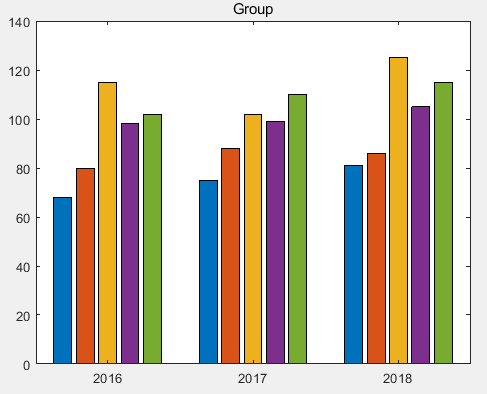2.1.2 直方图

绘制直方图的函数有两个：hist函数和rose函数。hist函数用于绘制直角坐标系下的直方图，rose函数用于绘制极坐标系下的直方图。

hist函数

调用格式：

• hist(y)
• hist(y, x)

通常，参数y是一个向量，绘图时将y中最小值和最大值之间的数值区间等分，并统计落在每个区间上元素的个数，然后以元素个数为高度，绘制条形图。当hist函数有两个输入参数时，第二个参数x用于指定区间的划分方式。当省略参数x时，默认采用10个等分区间。

close; clear; clc;
y = rand(500, 1);
subplot(121);
hist(y);
subplot(122);
hist(y, 25);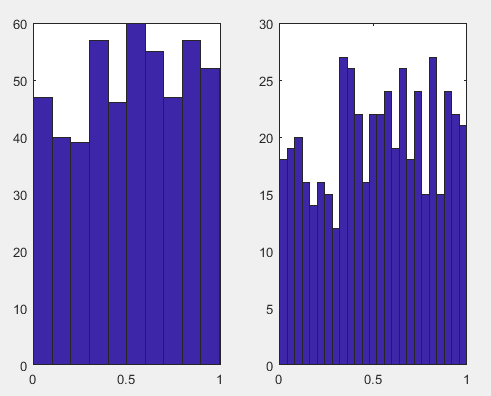rose函数

调用格式： rose(theta, x)

其中，参数theta是一个向量，用于确定每一区间与原点的角度（以弧度表示），选项x用于指定区间的划分方式。

举个例子。

close; clear; clc;
m = randn(500, 1);
theta = m * pi;
rose(theta);  % 默认为20个等分区间
title('在极坐标系下的直方图');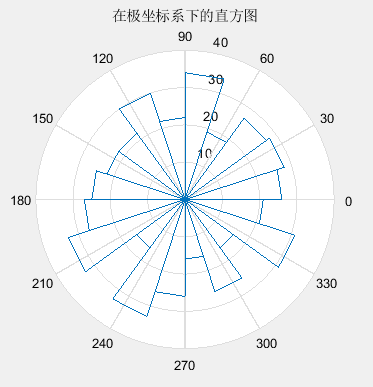2.2 面积类图形

2.2.1 扇形图

扇形图又称为饼图，采用函数pie()，其调用格式为：pie(x, explode)，其中，参数x存储待统计数据，选项explode控制图块的显示模式。

举例：某次考试优秀、良好、中等、及格、不及格的人数分别是5, 17, 23, 9, 4，采用扇形图做成绩统计分析。

close; clear; clc;
number = [5, 17, 23, 9, 4];
explode = [0, 0, 0, 0, 1];
pie(number, explode); % 从12点钟方向逆时针画图
legend('优秀', '良好', '中等', '及格', '不及格', 'location', 'eastoutside'); % location和eastoutside用于指定图例的位置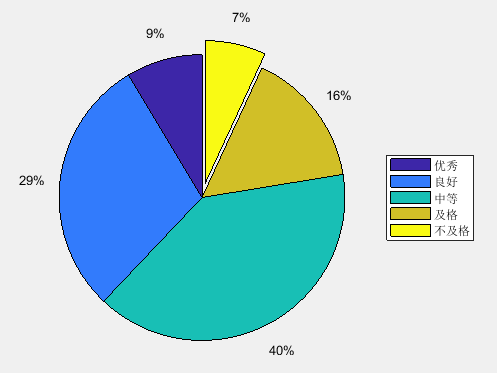2.2.2 面积图

采用函数area()。课程中未做介绍，用时再查了。

2.3 散点类图形

散点类图形常用于实验数据的表示，比较实验数据和理论值之间的差异。

• scatter函数：散点图
• stairs函数：阶梯图
• stem函数：杆图

三个函数的用法与plot函数相似。以scatter函数为例，说明这类函数的用法。

scatter函数

调用格式： scatter(x, y, 选项, 'filled')，参数x,y通常为同等长度的向量，用于定位数据点，选项的设置与plot函数相同，用于指定线型、颜色、数据点标记。如果数据点标记是圆圈，方块等，可以用filled填充数据点标记。省略filled时，数据点标记是空心的。

举个例子：以散点图形式绘制桃心曲线。

close; clear; clc;
t = 0 : pi/50 : 2*pi;
x = 16 * sin(t).^3;
y = 13*cos(t) - 5*cos(2*t) - 2*cos(3*t) - cos(4*t);
scatter(x, y, 'rd', 'filled'); % rd表示红色菱形，filled表示填充该菱形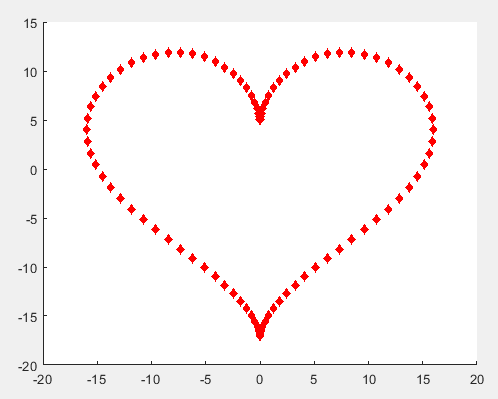2.4 矢量类图形

• compass函数：罗盘图
• feather函数：羽毛图
• quiver函数：箭头图

三个函数的用法与plot函数相似。常用quiver函数绘制矢量场，如磁力线等。箭头方向表示矢量方向，箭头长短表示矢量大小。

以quiver函数为例，说明矢量类图形的绘制方法。quiver函数的调用格式为：

quiver(x, y, u, v)，其中，(x,y)指定矢量的起点，(u,v)指定矢量的终点。x, y, u, v是同长度的向量或者同型的矩阵。若省略x, y，则在xy平面上均匀地取若干个点作为矢量的起点。

举个例子：已知向量A,B，求A+B，并用矢量图表示。

close; clear; clc;
A = [4, 5];
B = [-10, 0];
C = A + B;
hold on;
quiver(0, 0, A(1), A(2));
quiver(0, 0, B(1), B(2));
quiver(0, 0, C(1), C(2));
text(A(1), A(2), 'A');
text(B(1), B(2), 'B');
text(C(1), C(2), 'C');
axis([-12, 6, -1, 6]);
grid on;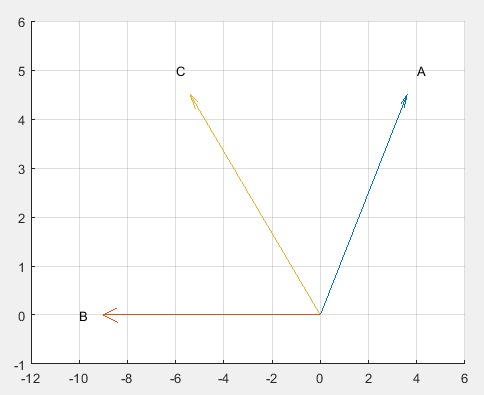------------------------------------------------------------------------------

四    三维曲线

最基本的三维曲线绘制函数是plot3函数。此外还有fplot3函数。

4.1 plot3函数

基本用法：plot3(x, y, z)，其中，参数x, y, z组成一组曲线的空间坐标。通常x, y, z是长度相同的向量。

举个例子：绘制一条空间折线。

close; clear; clc;
x = [0.2, 1.8, 2.5];
y = [1.3, 2.8, 1.1];
z = [0.4, 1.2, 1.6];
plot3(x, y, z);
grid on;
axis([0, 3, 1, 3, 0, 2]);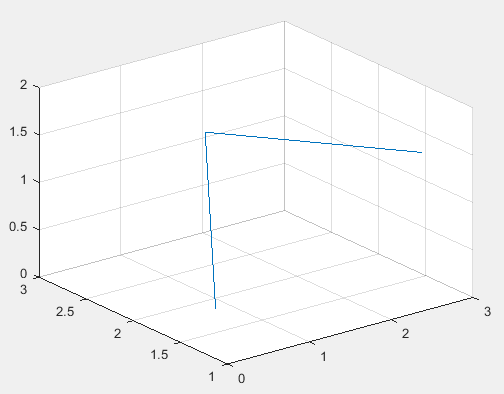再举个例子：绘制螺旋线。

close; clear; clc;
t = linspace(0, 10*pi, 200);
x = sin(t) + t.*cos(t);
y = cos(t) - t.*sin(t);
z = t;
subplot(121);
plot3(x, y, z);
grid on;
subplot(122);
plot3(x(1:4:200), y(1:4:200), z(1:4:200));
grid on;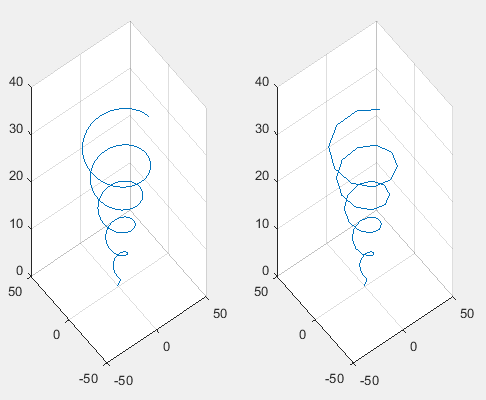plot3 函数参数的变化形式

通常情况下，plot3函数的参数x,y,z是等长的向量，但也会存在一些变化形式，如下：

• 参数x,y,z是同型矩阵时，则以矩阵x,y,z对应的列元素绘制空间曲线，曲线条数等于矩阵的列数。
• 参数x,y,z中有向量，也有矩阵时，向量的长度应与矩阵相符，也就是说行向量的长度应与矩阵的列数相同，列向量的长度应与矩阵的行数相同。

举个例子：在空间不同位置绘制5条正弦曲线。

close; clear; clc;
t = 0 : 0.01 : 2*pi;
t = t';
x = [t, t, t, t, t];
y = [sin(t), sin(t)+1, sin(t)+2,  sin(t)+3,  sin(t)+4];
z = [t, t, t, t, t];
plot3(x, y, z);
xlabel('x');
ylabel('y');
zlabel('z');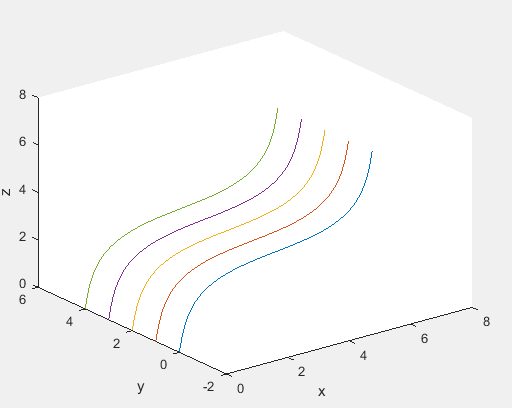含多组输入参数的plot3函数

调用格式： plot3(x1,y1,z1, x2,y2,z2, ..., xn,yn,zn)，每一组x,y,z向量构成一组数据点的空间坐标，绘制一条曲线。

举个例子：绘制3条不同长度的空间正弦曲线。

close; clear; clc;
t1 = 0 : 0.01 : 1.5*pi;
t2 = 0 : 0.01 : 2*pi;
t3 = 0 : 0.01 : 3*pi;
plot3(t1, sin(t1), t1, t2, sin(t2)+1, t2, t3, sin(t3)+2, t3);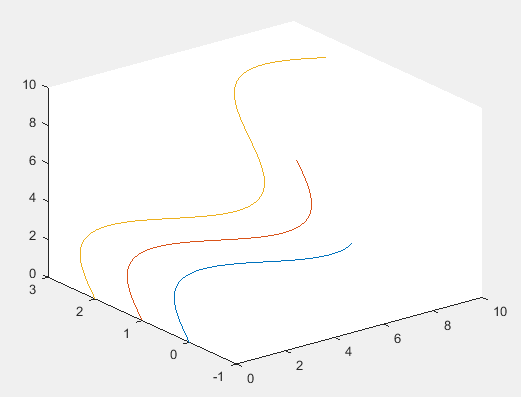含选项的plot3函数

调用格式：plot3(x, y, z, 选项)，选项用于指定曲线的线型、颜色和数据点标记。选项的用法与plot函数相同。

举个例子。

close; clear; clc;
t = 0 : pi/50 : 6*pi;
x = cos(t);
y = sin(t);
z = 2*t;
plot3(x, y, z, 'rp'); % rp表示红色五角星
xlable('X');
ylabel('Y');
zlabel('Z');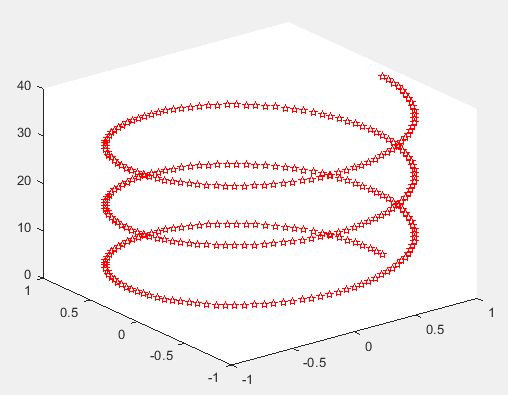4.2 fplot3函数

如果曲线的x,y,z坐标用参数方程定义，且参数方程只有一个自变量，则可以使用MATLAB提供的fplot3函数绘制三维曲线。

fplot3函数的基本调用格式为：fplot3(funx, funy, funz, tlims)

其中，funx, funy, funz表示定义曲线x, y, z坐标的函数，通常采用函数句柄的形式。tlims为参数函数自变量的取值范围，用二元向量[tmin, tmax]描述，默认值为[-5, 5]。

举个例子：绘制墨西哥帽顶曲线。

close; clear; clc;
x = @(t)exp(-t/10).*sin(5*t);
y = @(t)exp(-t/10).*cos(5*t);
z = @(t)t;
tlims = [-12, 12];
fplot3(x, y, z, tlims);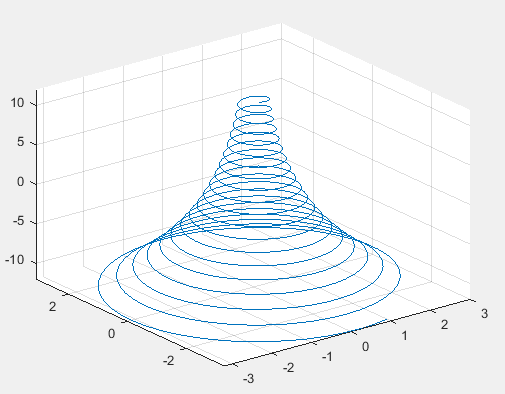在fplot3函数中，可以指定曲线的线型、颜色和数据点标记。调用格式为：fplot3(funx, funy, funz, tlims，选项)，其中，选项的设置与plot函数相同。

举个例子：将上述的墨西哥帽顶曲线用红色圆圈表示出来。

close; clear; clc;
x = @(t)exp(-t/10).*sin(5*t);
y = @(t)exp(-t/10).*cos(5*t);
z = @(t)t;
tlims = [-12, 12];
fplot3(x, y, z, tlims, 'ro');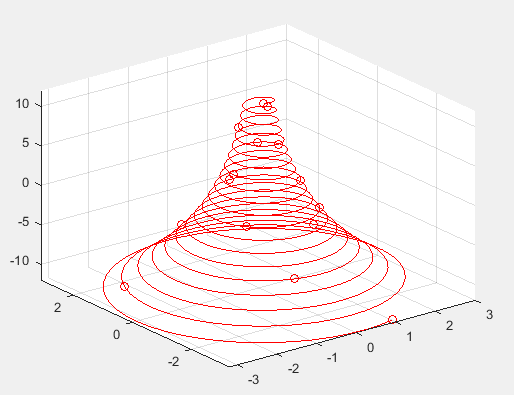------------------------------------------------------------------------------

五    三维曲面

通常，绘制三维曲面图之前要先生成平面网格数据，再调用mesh函数或surf函数绘制三维曲面。如果三维曲面是用含两个自变量的函数定义的，那么还可以使用fmesh函数或fsurf函数。

5.1 平面网格数据的生成

5.1.1 利用矩阵运算生成

例如。

close; clear; clc;
x = 2 : 6;
y = (3 : 8)';
X = ones(size(y)) * x
Y = y * ones(size(x))

其中，x=[2, 3, 4, 5, 6]，y=[3; 4; 5; 6; 7; 8]，生成的矩阵X和Y如下：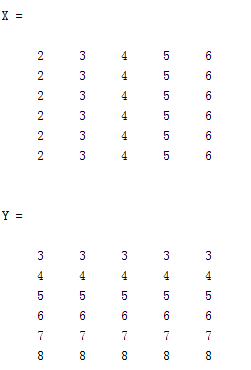矩阵X的每一行都是向量x，矩阵X的行数等于向量y的元素的个数。矩阵Y的每一列都是向量y，矩阵Y的列数等于向量x的元素的个数。矩阵X和矩阵Y都是6行5列，相同位置上的元素构成一个坐标点，这些坐标点表示平面网格上的点。如下图所示：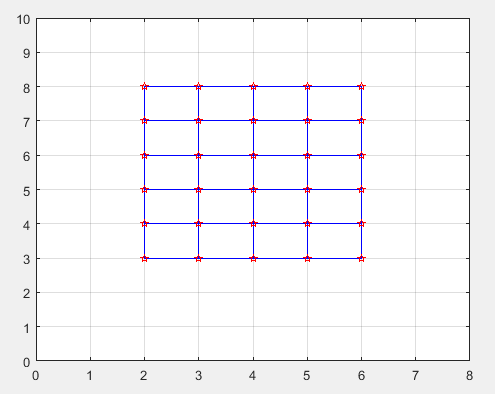5.1.2 利用meshgrid函数生成

调用格式为： [X, Y] = meshgrid(x, y)

其中，参数x,y为向量，存储网格点坐标的X,Y为矩阵。

例如，仍然是上例中的x,y，生成平面网格数据。

close; clear; clc;
x = 2 : 6;
y = (3 : 8)';
[X, Y] = meshgrid(x, y)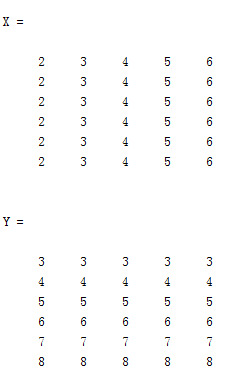与利用矩阵运算生成的结果相同。

5.2 绘制三维曲面的函数

mesh函数和surf函数的基本调用格式：

• mesh(x, y, z, c)：用于绘制三维网格图
• surf(x, y, z, c)：用于绘制三维曲面图

一般情况下，x, y, z是三个同型的矩阵。其中，x, y是网格坐标矩阵，z是网格点上的高度矩阵，c用于指定在不同高度下的曲面颜色。当c省略时，MATLAB认为c与z相同。

举个例子：绘制三维曲面图z=x*exp(-x²-y²)

close; clear; clc;
t = -2 : 0.2 : 2;
[X, Y] = meshgrid(t);
Z = X .* exp(-X.^2 - Y.^2);
subplot(131);
mesh(X, Y, Z);
subplot(132);
surf(X, Y, Z);
subplot(133);
plot3(X, Y, Z);
grid on; % 作用于最后一个子图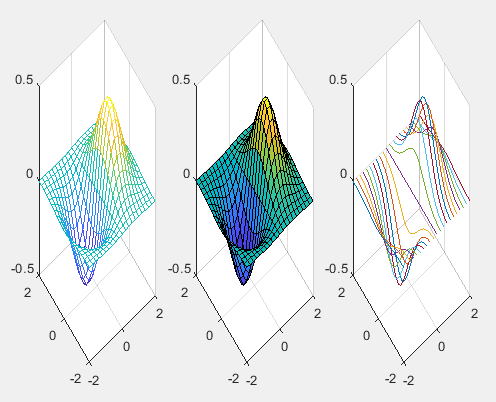mesh函数和surf函数的其他调用格式：

• mesh(z, c)
• surf(z, c)

当x,y省略时，z矩阵的第2维（列）下标当做x轴坐标，z矩阵的第1维（行）下标当做y轴坐标。

与mesh函数和surf函数相关的函数：

• 带等高线的三维网格曲面函数meshc
• 带底座的三维网格曲面函数meshz
• 具有等高线的曲面函数surfc
• 具有光照效果的曲面函数surfl

举个例子。

close; clear; clc;
x = 0:0.1:2;
y = 1:0.1:3;
[X, Y] = meshgrid(x, y);
Z = (X-1).^2 + (Y-2).^2 - 1;
subplot(221);
meshc(X, Y, Z);
title('meshc(X, Y, Z)');
subplot(222);
meshz(X, Y, Z);
title('meshz(X, Y, Z)');
subplot(223);
surfc(X, Y, Z);
title('surfc(X, Y, Z)');
subplot(224);
surfl(X, Y, Z);
title('surfl(X, Y, Z)');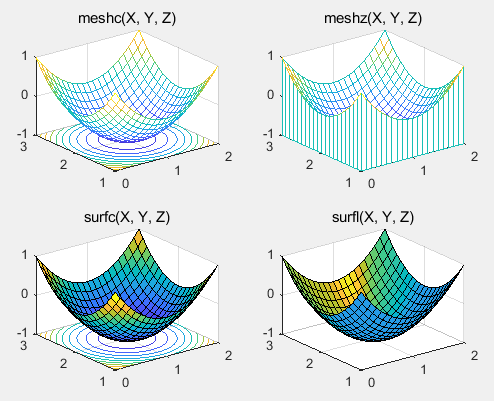5.3 标准三维曲面

(1) sphere函数：用于绘制三维球面

[x,y,z] = sphere(n)  % n表示球面的圆滑程度，默认值为20

(2) cylinder函数：用于绘制三维柱面

[x,y,z] = cylinder(R,n)

举个例子：绘制一个三维球面。

[x, y, z] = sphere(50);
surf(10*(x,y,z));
axis equal;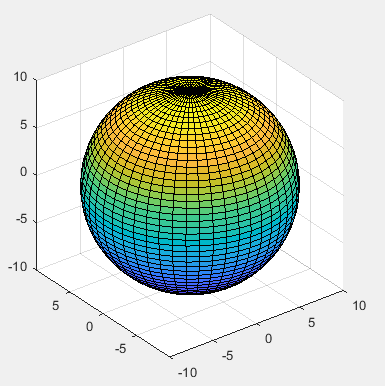举个例子：用cylinder函数分别绘制柱面、花瓶和圆锥面。

close; clear; clc;
subplot(131);
[x,y,z]= cylinder;
surf(x,y,z);
subplot(132);
t = linspace(0, 2*pi, 40);
[x,y,z] = cylinder(2+cos(t), 30);
surf(x,y,z);
subplot(133);
[x,y,z] = cylinder(0:0.2:2, 30);
surf(x,y,z);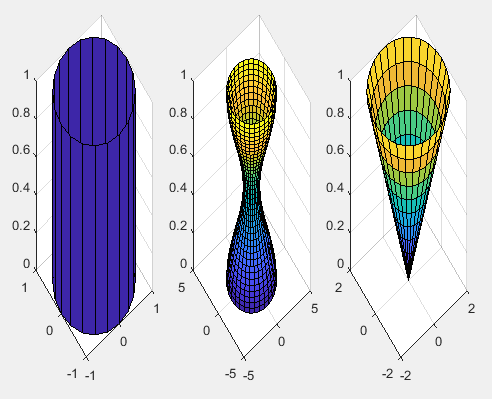5.4 fmesh函数和fsurf函数

如果三维曲面是用含两个自变量的函数定义的，那么还可以使用fmesh函数或fsurf函数。

调用格式为：

• fmesh(funx, funy, funz, uvlims)
• fsurf(funx, funy, funz, uvlims)

其中，funx, funy, funz表示定义曲面x, y, z坐标的函数，通常采用函数句柄的形式。uvlims为funx, funy, funz的自变量的取值范围，用四元向量[umin, umax, vmin, vmax]描述，默认为[-5, 5, -5, 5]。

举个例子：绘制螺旋曲面。

close; clear; clc;
funx = @(u,v)u.*sin(v);
funy = @(u,v)-u.*cos(v);
funz = @(u,v)v;
fsurf(funx, funy, funz, [-5, 5, -5, -2]);
hold on;
fmesh(funx, funy, funz, [-5, 5, -2, 2]);
hold off;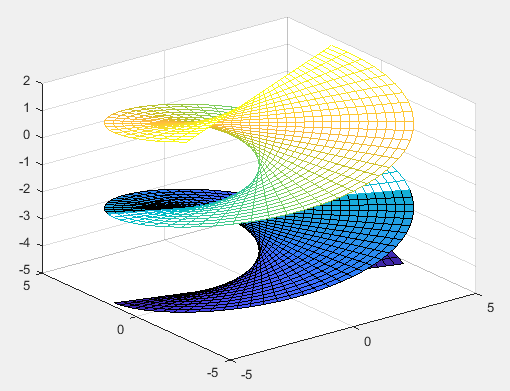------------------------------------------------------------------------------

六    图形修饰处理

图形修饰处理可以渲染和烘托图形的表现效果。主要包括视点处理，色彩处理，裁剪处理等。

6.1 视点处理

视点处理就是改变观察点的位置。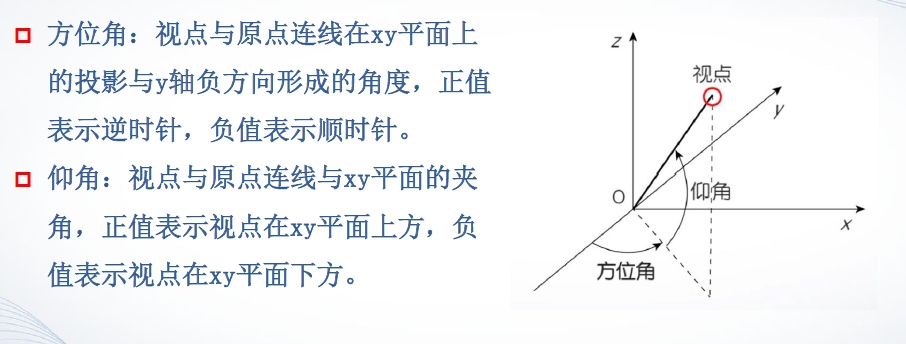view函数的基本用法：view(az, el)，其中az为方位角，el为仰角，它们均以度为单位。系统默认的视点方位角为-37.5°，仰角为30°。

举个例子：绘制一个曲面，并从不同视点观察曲面。

close; clear; clc;
[x,y] = meshgrid(0:0.1:2, 1:0.1:3);
z = (x-1).^2 + (y-2).^2 - 1;
subplot(2,2,1), surf(x,y,z);
title('方位角=-37.5°，仰角=30°');
subplot(2,2,2), surf(x,y,z);
view(0, 90); % 方位角设置为0°，仰角设置为90°
title('方位角=0°，仰角=90°');
subplot(2,2,3), surf(x,y,z);
view(90, 0); % 方位角设置为90°，仰角设置为0°
title('方位角=90°，仰角=0°');
subplot(2,2,4), surf(x,y,z);
view(-45, -60); % 方位角设置为-45°，仰角设置为-60°
title('方位角=-45°，仰角=-60°');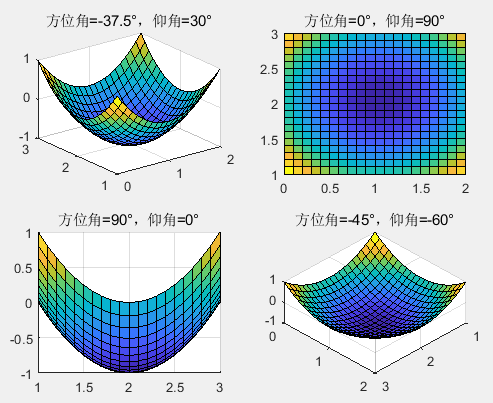view函数的其他用法：

• view(x,y,z)   % (x,y,z)为视点在笛卡尔坐标系中的位置
• view(2)   % 设置从二维平面观察图形，即方位角为0°，仰角为90°
• view(3)  %  设置从三维空间观察图形，视点使用默认的方位角和仰角

6.2 色彩处理

很多时候一个简单的二维或三维图形并不能显示出想要的全部信息，这时候颜色可以使图形呈现更多的信息。

6.2.1 颜色的向量表示

向量表示方法： [R, G, B]    % R-红，G-绿，B-蓝

向量的三个元素均在[0,1]范围内取值，3个元素依次表示红，绿，蓝3种颜色的相对亮度，称为RGB三元组。例如：[0,0,1]表示蓝色，[1,0,0]表示红色，[0,1,0]表示绿色，[1,1,1]表示白色，[0,0,0]表示黑色。

6.2.2 色图(Colormap)

色图矩阵是一个m×3的矩阵，它的每一行是RGB三元组，m行表示该色图包含了m种颜色。MATLAB的内建色图包括冷暖色图，四季色图，灰度色图等，内建色图是一个64×3的矩阵。MATLAB（2016a）默认的色图是parula。

内建色图主要有：指定当前图形使用的色图

语法形式：

• colormap cmapname   % 其中cmapname是内建色图名，如parula, jet, hsv等等，参照上图
• colormap(cmap)    % cmap表示色图矩阵

举个例子。

采用如下代码，绘制尖峰函数曲面，系统默认的色图是parula

surf(peaks);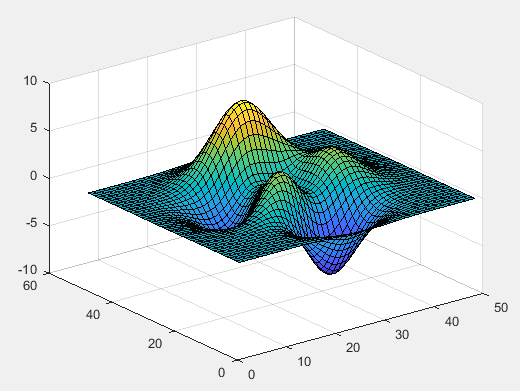若要使用hot色图，代码如下

surf(peaks);
colormap hot;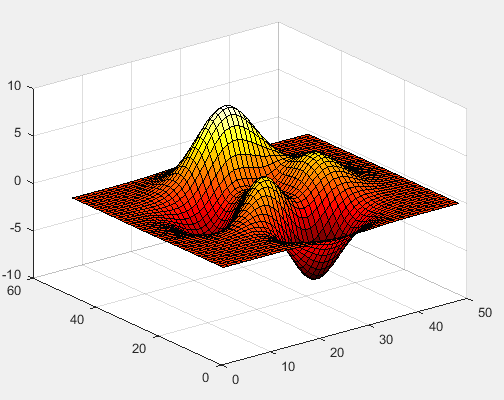创建色图矩阵

色图矩阵的每一行是RGB三元组，可以自定义色图矩阵，也可以调用MATLAB提供的函数来定义色图矩阵。

例如：创建一个灰色系列色图矩阵。

close; clear; clc;
c = [0, 0.2, 0.4, 0.6, 0.8, 1]';
cmap = [c, c, c];
surf(peaks);
colormap(cmap);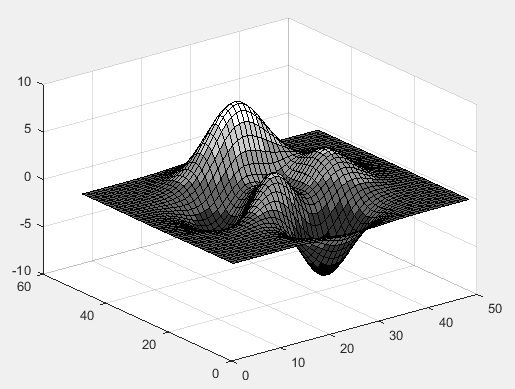6.2.3 三维图形表面的着色

举个例子：使用同一色图，以不同着色方式绘制圆锥体。

close; clear; clc;
[x,y,z] = cylinder(pi : -pi/5 : 0, 10);
colormap(lines);
subplot(131);
surf(x,y,z);
subplot(132);
surf(x,y,z);
subplot(133);
surf(x,y,z);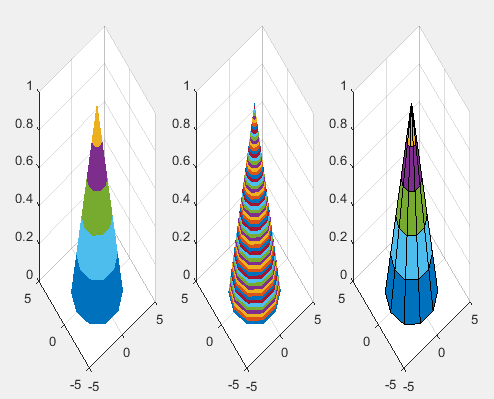6.3 裁剪处理

将图形中需要裁剪的部分对应的函数值设置为NaN，这样在绘制图形时，函数值为NaN的部分将不显示出来，从而达到对图形进行裁剪的目的。

举个例子：绘制3/4圆。

close; clear; clc;
t = linspace(0, 2*pi, 100);
x = sin(t);
y = cos(t);
subplot(121), plot(x,y);
axis([-1.1, 1.1, -1.1, 1.1]);
axis square;
subplot(122);
p = y>0.5; % p是一个1×100的向量，y>0.5时相应位置取1，否则取0。
y(p) = NaN;
plot(x,y);
axis([-1.1, 1.1, -1.1, 1.1]);
axis square;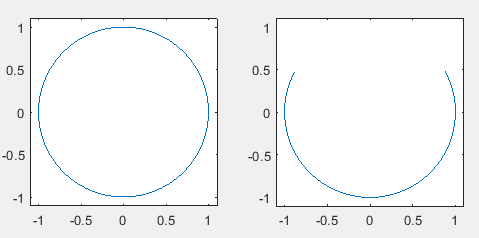再举个例子：绘制3/4球面。

close; clear; clc;
[x,y,z] = sphere(60); % 60表示圆滑程度
p = z>0.5;
z(p) = NaN;
surf(x,y,z);
axis([-1, 1, -1, 1, -1, 1]);
axis equal;
view(-45, 30);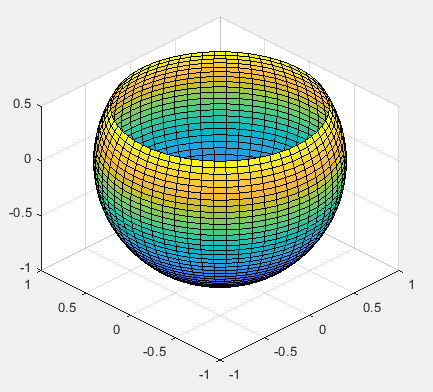-----------------------------------------------------------------------------

七    交互式绘图工具

快速构建图形与调整图形效果。MATLAB交互式绘图工具包括：“绘图”选项卡、图形窗口绘图工具、图形窗口菜单和工具栏。

7.1 “绘图”选项卡

“绘图”选项卡位于MATLAB主窗口功能区中，如下图。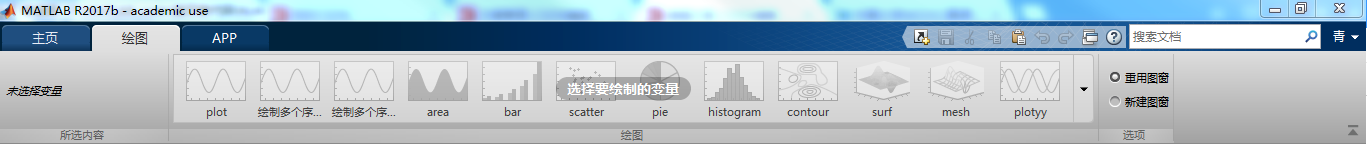当在工作空间中新建一个变量之后，比如建立了一个变量x。选中工作空间中变量x之后，在“所选内容”栏将会出现变量x，同时“绘图”栏的绘图命令变量，处于可用状态，点击不同的绘图命令可显示不同的图形。右侧的“选项”栏，“重用图窗”表示下一次绘制的图形将覆盖当前的图形窗口，“新建图窗”表示当前图窗不会被覆盖，下一次绘制的图形会在新建的图窗中显示出来。

7.2 图形窗口绘图工具

绘制图形后，如果需要修改绘图参数或者显示方式，可利用MATLAB的图形窗口提供的绘图工具。

7.2.1 显示绘图工具

• 采用“显示绘图工具和停靠图形”按钮，如下图：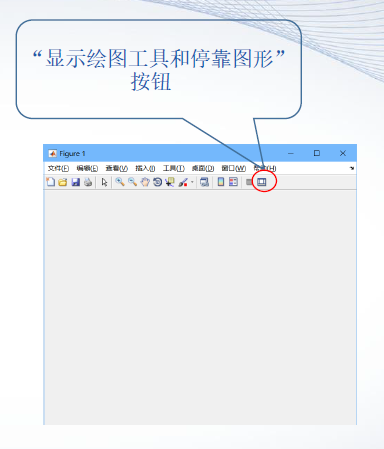• 命令行窗口中输入命令：>>plottools

7.2.2 绘图工具的组成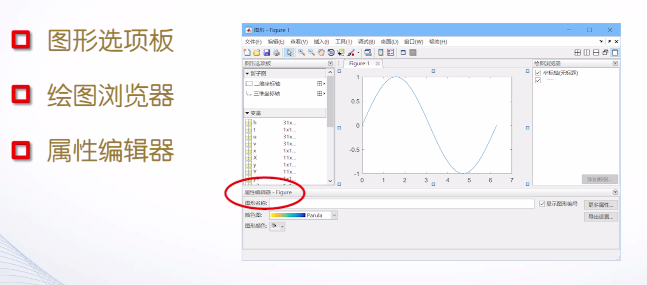图形选项板：用于在图形窗口中添加和排列子图，观察和选择绘图数据以及添加图形标注。

7.3 图形窗口菜单和工具栏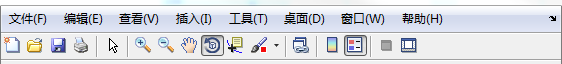展开全文• matlab画图橙色代码从Matlab创建Ti K Z / pgf图形 tikzfig是Matlab工具箱，可使用来以编程方式创建pdf格式的图形文件。 它将Ti k Z接口的一部分作为Matlab API公开给pgf，以便图形语句导致构建LaTeX文件，该文件透明...
• MATLAB画图

千次阅读 2020-02-06 17:32:43
有不少研究者都采用MATLAB进行绘图，因为MATLAB画图功能齐全又不失美观，接下来我将结合具体代码记录一下MATLAB画图的一些操作和心得。 第一段代码： %Fig1. close all clear syms w p c t d pe b we a c=1; d=1; b...matlab
• 1.在命令窗口输入命令时，可以不必每输入一条命令就按enter键执行，...2.如何将数据文件导入matlab中并画图：点击file中import data,然后就可以导入文件，然后再workspace里面，点击该数据文件，就可以出现以下图...
• matlab画图参数

2021-04-20 15:20:50
画图函数句柄的使用 每个画图函数含有的输入参数是很多的, 然而我们在看函数的帮助(help)的时候,所 能得到的只是一些基本的......数学建模与数学实验 MATLAB作图后勤工程学院数学教研室 后勤工程学院数学教研室 二维...
• matlab画图特殊符号

千次阅读 2021-04-24 20:20:09
MATLAB 中使用 LaTex 字符 1.Tex 字符表 在 text 对象的函数中(函数 title、xlabel、ylabel、zlabel 或 text), 说明文字除使用标准的 ASCII 字符外,还可......matlab特殊字符_工学_高等教育_教育专区。本文说明了...
• MATLAB论文绘图模板与尺寸设置MATLAB图形绘制尺寸设置MATLAB常用指令参考文献新的改变功能快捷键合理的创建标题，有助于目录的生成如何改变文本的样式插入链接与图片如何插入一段漂亮的代码片生成一个适合你的列表...matlab
• MATLAB画图的ppt-MaTLAB画图.rar 分享：MATLAB画图基础讲义。 第一页介绍： matlab语言的绘图功能 • 不仅能绘制几乎所有的标准图形， 而且其表现形式也是丰富多样的。 • matlab语言不仅具有高层绘图能 ...matlab
• 求大神教教菜鸟，如何在VS2010 中导入matlab，...1.如何调入MATLAB 画图功能到VS 中。 2.如何将 VS 中计算出来的数据传值到MATLAB中， 或是直接由MATLAB 读取数据库中数据进行计算然后画图展示。这两种方式哪种更简单，matlab
• Brain Connectivity Toolbox 是一个MATLAB工具箱
• matlab画图点的形状

千次阅读 2021-04-22 04:02:08
Matlab 绘图和坐标操作引自:http://blog.sciencenet....MATLAB作图青岛科后技勤大工学程学数院理数学学教院研数室学系 二维图形 三维图形 图形处理 特殊二、三维图形 实例 作业 1... 画图:用 matlab 二维绘图命令 pl...
• 使用matlab绘图函数plot绘图时系统默认设置了一些属性，例如坐标轴字号大小等并根据情况自动设置坐标轴显示的上下限，这些属性可以通过函数灵活改动，此处总结一小部分。figure;%设置坐标轴线宽,加边框,坐标字号...
• Matlab是一款强大的软件，画图功能使用方便，只需要简单的一个指令即可完成其他软件几十行甚至上百行代码才能实现的功能。这里就给初学者介绍一下简单的画图功能matlab画图
• 自动化专业综合设计报告 自动化专业综合设计报告 设计题目 利用MATLAB编程实现动态画图功能 所在实验室 自动化系统仿真实验室 指导教师 学生姓名 班级 计082-2 班 学号 200825502210 撰写时间 2012-3-1 成绩评定 一...
• matlab画图入门教程

千次阅读 2021-02-01 13:21:47
**matlab画图：**图像是数据结果的一种可视化表现，它能直观的体现你的数据结果，并且能体现你获得结果的准确性，在当前的大数据时代，在做数据分析的时候，将其可视化可以直观多维的展示数据，可以让人们更好的发现...matlab 数学建模
• 数学建模之MATLAB画图汇总

万次阅读 多人点赞 2018-08-21 12:21:38
Matlab提供了一些绘图选项，用于确定所绘曲线的线型、颜色和数据点标记符号。这些选项如表所示： 线型 颜色 标记符号 -实线 b蓝色 .点 s方块 :虚线 g绿色 o圆圈 ...
• matlab绘图-第5章 Matlab绘图功能-文本.doc matlab 绘图matlab
• p=plot(1:1:100, mean(reshape(sum(QoE4(1:129000),2),1290,[])), '-o','color',[255,0,51]/255,'linewidth', 1.5); p.MarkerSize = 5; %设置marker大小 p.MarkerIndices = 1:10:100; %设值marker个数matlab python
• Matlab画图插入Latex公式

万次阅读 多人点赞 2019-09-17 10:19:02
Latex语言是现在使用最广泛的Tex格式，Tex这种语言具有简单排版和程序设计的功能。 不过，LaTeX比TeX更为结构化，它有很多预设模版，包含供建立索引、表格、列表等的宏和公用软件。利用Latex，即使我们不会排版和...matlab latex 数学建模 数学建模国赛matlab
• matlab画图

2013-09-27 22:29:27
很好的资源，强大的绘图功能Matlab的特点之一，Matlab提供了一系列的绘图函数，用户不需要过多的考虑绘图的细节，只需要给出一些基本参数就能得到所需图形，这类函数称为高层绘图函数。此外，Matlab还提供了直接对...matlab
• Matlab 绘图 强大的绘图功能Matlab 的特点之一,Matlab 提供了一系列的绘图函数,用户不需要过多 的考虑绘图的细节, 只需要给出一些基本参数就能得到所需图形, ......MATLAB绘图函数_计算机软件及应用_IT/计算机_...
• 变换您的MATLAB绘图以适合黑暗模式主题背景！ 该功能测试哪些图形颜色不适合在深色背景上使用（通过颜色对比），并使用去饱和度和亮度方法相应地调整颜色。 此外，它还转换了相关的 Matlab 图形属性，例如轴和文本...matlab
• 标准 MATLAB plot() 函数只能生成不透明图。 plot_transparently 通过使用 patch() 而不是 plot() 绘制曲线来规避这个缺点。 它支持与 plot() 一起使用的大多数法线系列属性。 如果您需要更多属性，将它们添加到代码...matlab...

matlab画图功能matlab 订阅